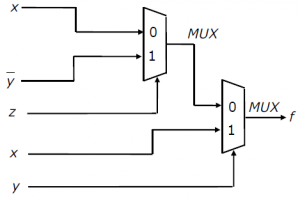# GATE | GATE-CS-2006 | Question 85Consider the circuit above. Which one of the following options correctly represents f (x, y, z)?
(A) xz’ + xy + y’z
(B) xz’ + xy + (yz)’
(C) xz + xy + (yz)’
(D) xz + xy’ + y’z

Explanation: Output from MUX 1=> Z’X+ZY’

Output from MUX2=> Y’(Z’X+ZY’)+YX

=>Y’Z+Y’Z’X+YX

=>Y’Z+X(Y’Z’+Y)

=>Y’Z+X(Y+Z’)        USING A+A’B=(A+B)

=>Y’Z+XY+XZ’

So Ans is (A).

Quiz of this Question